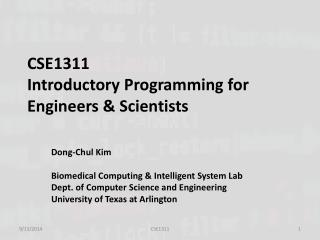DownloadDownload PresentationCSE1311 Introductory Programming for Engineers & Scientists

# CSE1311 Introductory Programming for Engineers & Scientists

Download Presentation## CSE1311 Introductory Programming for Engineers & Scientists

- - - - - - - - - - - - - - - - - - - - - - - - - - - E N D - - - - - - - - - - - - - - - - - - - - - - - - - - -
##### Presentation Transcript

1. CSE1311Introductory Programming for Engineers & Scientists Dong-Chul Kim Biomedical Computing & Intelligent System Lab Dept. of Computer Science and Engineering University of Texas at Arlington CSE1311

2. Lecture 1 Preliminary CSE1311

3. What is Programming? • What is computer programming? • Representation of a task or algorithm in a computer language. • What is an algorithm? • A set of directions for accomplishing a task. • Example: Turning a page by hand: • Lift hand. • Move hand to right side of book. • Grasp top-right corner of page. • Move hand from right to left until page is positioned so that you can read what is on the other side. • Let go of page. CSE1311

4. Algorithm • Another Example of an Algorithm • The algorithm for calculating the arithmetic mean (i.e., the average) of a set of numbers is • add all the numbers together • divide this sum by the quantity of numbers • Levels of Abstraction • Suppose a student is asked to come to the front of the class • high-level – stand, walk to front • mid-level – stand, turn 90◦ to the right, walk 15 feet, turn 90◦ to the left, walk 10 feet • low-level – contract specific muscles in a specific order • lowest-level – you think about walking, initiating many electrochemical reactions CSE1311

5. Low Level Language • Assembly • C (mid-level) CSE1311

6. Lowest Level CSE1311

7. C programming language • The C language was created in the early 1970s. The version we will learn is C89 (sometimes referred to as C90), which is based on the 1989 ANSI standard. • Why learn a 20 year old version of the language? • Because it is still the most common version. • High level / Mid level? • Starcraft? Warcraft? CSE1311

8. C programming language • Example CSE1311